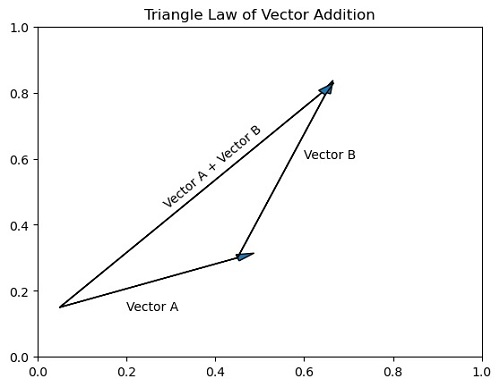# Python | Triangle Law of Vector Addition (Matplotlib Arrow Example)

Here, we are going to learn about the triangle law of vector addition (matplotlib arrow example) and its Python implementation.
Submitted by Anuj Singh, on August 04, 2020

There is a law of vector addition in physics, that is commonly known as the triangular law of vector addition and in this article, we are going to draw the vector diagram for the same using arrows in python. This example illustrates one of the very common and good examples of applications of arrows in real life.

```#Vector A

#Vector B

#Sum of the Vectors as A + B
```

Illustration:## Python code for triangle law of vector addition (matplotlib arrow example)

```import matplotlib.pyplot as plt

plt.figure()
plt.text(0.2, 0.14, 'Vector A')

plt.text(0.6, 0.6, 'Vector B')

plt.text(0.28, 0.45, 'Vector A + Vector B', rotation=40)

plt.show()

```

Output:

```Output is as figure
```

Languages: » C » C++ » C++ STL » Java » Data Structure » C#.Net » Android » Kotlin » SQL
Web Technologies: » PHP » Python » JavaScript » CSS » Ajax » Node.js » Web programming/HTML
Solved programs: » C » C++ » DS » Java » C#
Aptitude que. & ans.: » C » C++ » Java » DBMS
Interview que. & ans.: » C » Embedded C » Java » SEO » HR
CS Subjects: » CS Basics » O.S. » Networks » DBMS » Embedded Systems » Cloud Computing
» Machine learning » CS Organizations » Linux » DOS
More: » Articles » Puzzles » News/Updates